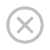# ASP.NET Razor - VB 逻辑条件

## If 条件

VB 允许您执行基于条件的代码。

• if 语句启动代码块
• 条件位于 if 和 then 之间
• 如果条件为真，则执行 if ... then 与 end if 之间的代码

### 实例

```@Code
Dim price=50
End Code
<html>
<body>
@If price>30 Then
@<p>The price is too high.</p>
End If
</body>
</html>
```

## Else 条件

if 语句能够包含 else 条件

else 条件定义条件为 false 时执行的代码。

### 实例

```@Code
Dim price=20
End Code
<html>
<body>
@if price>30 then
@<p>The price is too high.</p>
Else
@<p>The price is OK.</p>
End If
</body>
</htmlV>
```

## ElseIf 条件

### 实例

```@Code
Dim price=25
End Code
<html>
<body>
@If price>=30 Then
@<p>The price is high.</p>
ElseIf price>20 And price<30
@<p>The price is OK.</p>
Else
@<p>The price is low.</p>
End If
</body>
</html>
```

## Select 条件

select 代码块可用于测试一系列具体的条件：

### 实例

```@Code
Dim weekday=DateTime.Now.DayOfWeek
Dim day=weekday.ToString()
Dim message=""
End Code
<html>
<body>
@Select Case day
Case "Monday"
message="This is the first weekday."
Case "Thursday"
message="Only one day before weekend."
Case "Friday"
message="Tomorrow is weekend!"
Case Else
message="Today is " & day
End Select
<p>@message</p>
</body>
</html>
```

"Select Case" 之后是测试值 (day)。每个具体的测试条件以 case 关键词开头，其后允许任意数量的代码行。如果测试值匹配 case 值，则执行代码行。

select 代码块可为其余的情况设置默认的 case (default:)，允许在所有 case 均不为 true 时执行代码。